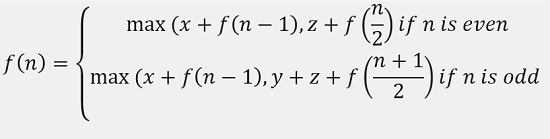Q:

# Minimum Time to Display N Character

You need to display N similar characters on a screen. You are allowed to do three types of operation each time.

1. You can insert a character,
2. You can delete the last character
3. You can copy and paste all displayed characters. After copy operation count of total written character will become twice.

All the operations are assigned different times.

• Time for insertion is X
• Time for deletion is Y
• Time for copy, paste is Z

You need to output minimum time to display N characters on the screen using these operations.

Input:

The first line of input contains an integer T denoting the number of test cases. Then T test cases follow. Each test case contains an integer N denoting the number of same characters to write on the screen. The next line contains time for insertion, deletion, and copying respectively.

Output:

Print the minimum time to display N characters on the screen.

Constraints:

``` 1 <= T <= 100
1 <= N <= 100
1 <= X, Y, Z <= N
```

Example:

```Input:
Test case: 2
First test case,
N, number of characters to be displayed = 9
Value of X, Y, Z respectively,
1 2 1
N, number of characters to be displayed = 10
Value of X, Y, Z respectively,
2 5 4

Output:
minimum time for first test case: 5
minimum time for second test case: 14
```

Explanation:

```For the first test case no of character to be displayed is: 9
Time for insertion is 1
Time for deletion is 2
Time for copy paste is 1
Say the similar character is 'a'
So the best way is
Insert two character
Time is 2
Copy paste
Total character so far is 4
Total time 3
Copy paste again
Total character so far is 8
Total time 4
Insert gain
Total character so far is 9
Total time 5
This is the most optimum way we can solve this```

This is a recursive problem

And we have a few possibilities,

1. Simply insert
2. Copy-paste
3. Delete

Now we need to understand the optimal option based on the situation

Say a situation where we need to reach character 13 and we are at character 7 displayed already

Also, Cost of Inserting 6 digits is much higher than one-time copy paste and deleting ( just consider this case, it may not happen always if copy paste option is costly)

So, the question is when the "delete" options is useful

It's useful for such case what I mentioned. So if we formulate the recursion

Say,N = number of characters to be displayed.

So, if n is odd only then we need deletion if n is we even don't need that.

Now, we have our recursion, so we can write the recursive function. Since there will be many overlapping sub-problems, we can wither use top-down DP or bottom-up DP. I have used memoization in my implementation. As an exercise, you should be able to convert the above recursion to tabulation DP.

C++ Implementation:

``````#include <bits/stdc++.h>
using namespace std;

int dp;

int minimum(int a, int b)
{
return a > b ? b : a;
}

int my(int cur, int x, int y, int z)
{

if (cur == 1) {

return x;
}
// meoization, dont compute what's already computed
if (dp[cur] != -1)
return dp[cur];
if (cur % 2 == 0) { //for even n

dp[cur] = minimum(x + my(cur - 1, x, y, z), z + my(cur / 2, x, y, z));
}
else { //for odd n

dp[cur] = minimum(x + my(cur - 1, x, y, z), z + y + my((cur + 1) / 2, x, y, z));
}
return dp[cur];
}

int main()
{
int t, n, item;

cout << "enter number of testcase\n";
cin >> t;

for (int i = 0; i < t; i++) {
cout << "Enter number of characters\n";
cin >> n;

int x, y, z;

cout << "Insert time for insertion, deletion and copy respectively\n";
cin >> x >> y >> z;

for (int i = 0; i <= n; i++)
dp[i] = -1;

cout << "Minimum time is: " << my(n, x, y, z) << endl;
}

return 0;
}``````

Output:

```enter number of testcase
3
Enter number of characters
8
Insert time for insertion, deletion and copy respectively
3 2 5
Minimum time is: 16
Enter number of characters
8
Insert time for insertion, deletion and copy respectively
1 1 3
Minimum time is: 7
Enter number of characters
3
Insert time for insertion, deletion and copy respectively
1 4 6
Minimum time is: 3```